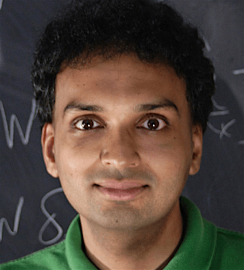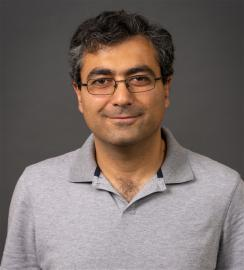Number theory is concerned with the integers and rational numbers, and objects built out of them and related to them. Questions asked in number theory include "Can one solve a given system of polynomial equations with rational coefficients in rational numbers?" and "How are the prime numbers distributed?"

Number theory includes many famous questions, both solved and unsolved. For example, Fermat's Last Theorem (that there are no nontrivial integer solutions to x^n + y^n = z^n, with n > 2) is a famous result in number theory, due to Andrew Wiles. Famous open questions in number theory include the Birch and Swinnterton-Dyer conjecture, the Riemann Hypothesis, and Goldbach's conjecture.

Modern number theory uses techniques from and contributes to areas across mathematics, including especially representation theory and algebraic geometry. Number theory also plays an important role in computer science, especially in public-key cryptography.

### Faculty##### Alina Bucur

Research Areas

Coxeter Groups

Kac-Moody Algebras

Metaplectic Forms

Automorphic Forms##### Kiran Kedlaya

Research Areas

Arithmetic Algebraic Geometry##### Cristian Popescu

Research Areas

Algebraic Number Theory

Arithmetic Geometry##### Alireza Salehi Golsefidy

Research Areas

Number Theory

Combinatorics

Arithmetic lattices

Homogeneous dynamical systems##### Claus Sorensen

Research Areas

Number Theory

Langlands program